# Part 4: Geometric shape analysis¶

In the previous notebooks, we presented the main tools that can be used to process bitmap images: convolutions, thresholdings, subsamplings and erosions/dilations. Now, it's time to go beyond these low-level tools to do some geometry!

But first, we need to re-import standard modules and re-define our display routine:

In :
%matplotlib inline
import center_images             # Center our images
import matplotlib.pyplot as plt  # Graphical display
import numpy as np               # Numerical computations

def display(im):  # Define a new Python routine
"""
Displays an image using the methods of the 'matplotlib' library.
"""
plt.figure(figsize=(8,8))                    # Create a square blackboard
plt.imshow(im, cmap="gray", vmin=0, vmax=1)  # Display 'im' using gray colors,
#     from 0 (black) to 1 (white)


Our "test image" is the axial slice introduced in the previous notebook:

In :
I = imread("data/aortes/1.jpg", as_gray=True)  # Import a jpeg image as a grayscale
I = I / 255  # For convenience, we normalize the intensities in the [0,1] range
display(I)   # Let's display our slice: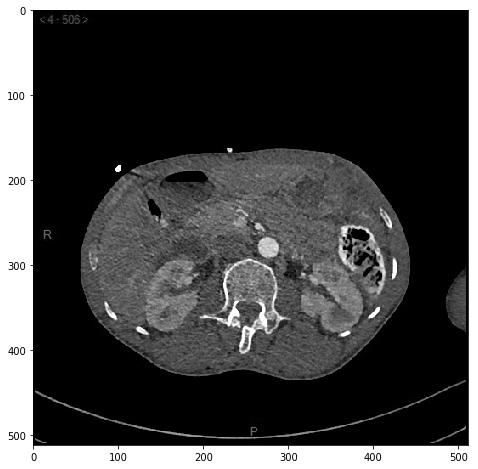We segment the aorta using thresholdings, erosions and dilations:

In :
from scipy.ndimage.morphology import binary_erosion, binary_dilation

mask   = (I > .6) & (I < .9)                   # Threshold the image values
core   = binary_erosion( mask, iterations=5)   # Remove the thin components
region = binary_dilation(core, iterations=10)  # Core growth -> trust region
aorta  = (I > .6) & region  # Intersection between "image is not too dark"
#                        and the trust region

display(aorta + .5*I)  # Display the segmented aorta, with the slice in the background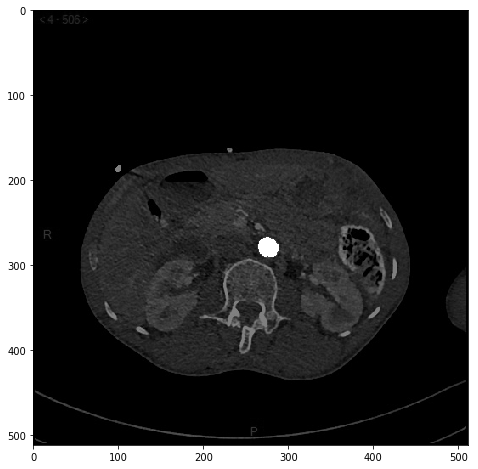### Working with point clouds¶

What do we do now? Ideally, we'd like to extract geometric descriptors from this binary mask: position, width, height, etc. To access the coordinates of the pixels that are labeled as "being part of the aorta", we make use of the nonzero() method provided by the numpy library:

In :
def extract_points(mask) :
"""
Turns a binary mask (bitmap) into a list of point coordinates (an (N,2) array).
"""
return np.vstack(mask.nonzero()).astype(float).T[:,::-1]  # Dark magic with NumPy...


As we apply it to our mask aorta, which is equal to 1 on the vessel and 0 elsewhere, we end up with a 2D array that can be understood as a list of points:

In :
y = extract_points(aorta)  # y is a "point cloud" representation of the aorta

print("Shape of the point cloud array - (lines,columns):", y.shape)

Shape of the point cloud array - (lines,columns): (454, 2)


The computation above shows that our aorta is made up of 454 points in 2D, the y[j]'s, whose coordinates can be accessed through standard indexing operations:

In :
print("Coordinates of the tenth point:", y)
print("Abscissa of the third point:", y[2,0])
print("Ordinate of the last point:", y[-1,1])

Coordinates of the tenth point: [276. 268.]
Abscissa of the third point: 276.0
Ordinate of the last point: 290.0


The full array can be displayed as text:

In :
print("Points' positions:")
print(y)

Points' positions:
[[274. 267.]
[275. 267.]
[276. 267.]
[277. 267.]
[271. 268.]
[272. 268.]
.    .
.    .
[282. 290.]
[283. 290.]]


Or as a set of small disks, using the scatter(...) routine:

In :
plt.figure(figsize=(10,10))  # Create a square blackboard
plt.scatter(y[:,0], y[:,1], s=9, c="red")  # Use red dots of size 9
plt.imshow(I, cmap="gray")   # Display the slice in the background
plt.axis([240,310,240,310])  # Crop the view around the aorta
plt.gca().invert_yaxis()     # Invert the "y" axis, according to the "image" convention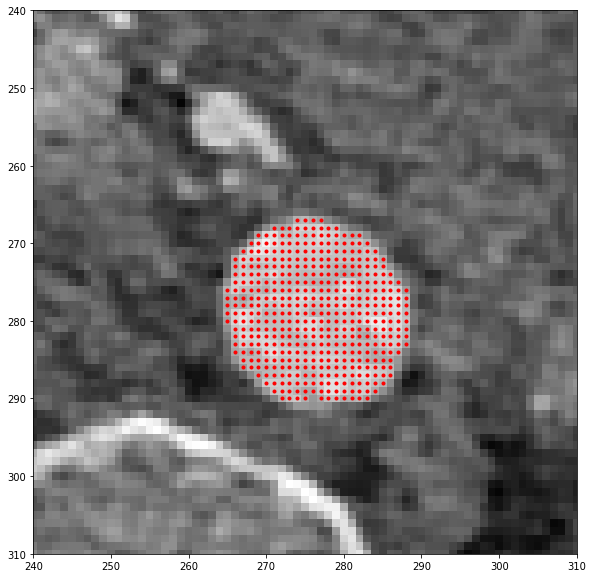### Basic operations on point clouds¶

Even though aorta (mask) and y (point cloud) are two arrays of numbers which encode the same information, they do so in a completely different way:

• aorta has a fixed size (512x512). It is the result of a local processing on bitmaps (our rudimentary "Convolutional Neural Network" for segmentation) and can be used as a look-up table to compute a Dice coefficient. Unfortunately though, it is impractical to work with: the shape's coordinates are implicitely encoded and cannot be manipulated easily.

• y has a fixed number of columns (2), but its number of rows depends on the surface of the segmented region. Crucially, the aorta's geometry is now explicit: simple mathematical operations will allow us to move and stretch the vessel without hassle.

As taught in middle school, additions on y encode translations and allow us to move around this aorta:

In :
w = y + [-3.5, 0]  # Translation to the left
z = y + [20, -10]  # Translation to the upper right (the y axis points to the bottom)

plt.figure(figsize=(10,10))                  # Create a square blackboard
plt.scatter(y[:,0], y[:,1], s=9, c="red")    # Display'y' using red   dots of size 9
plt.scatter(w[:,0], w[:,1], s=9, c="blue")   # Display'w' using blue  dots of size 9
plt.scatter(z[:,0], z[:,1], s=9, c="orange") # Display 'z' using orange dots of size 9
plt.imshow(I, cmap="gray")                   # Display the slice in the background
plt.axis([240,310,240,310])                  # Crop the view around the aorta
plt.gca().invert_yaxis()     # Invert the "y" axis, according to the "image" convention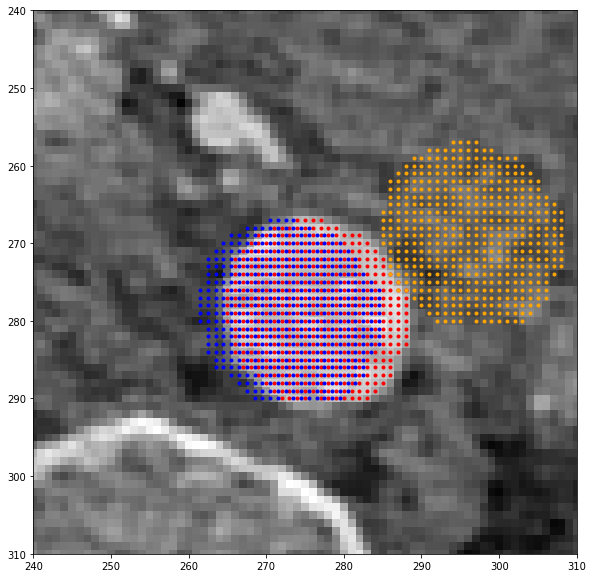### Creating a template shape¶

When performing (computational) anatomy, we'd like to compare the subject's shape to a reference template image. In this super-simple toy example, we will compare the segmented aorta y to a model ellipse x, generated as a deformation of a unit disk x_0. Let us simply recall that an ellipse of center $(a,o)$, width $w$ and height $h$ is a set of equation

\begin{align} (\text{Ellipse})~:~\frac{(x-a)^2}{w^2 / 4}~+~ \frac{(y-o)^2}{h^2 / 4} ~=~ 1 , \end{align}

that can be generated through the translation and stretching of a disk.

To build a disk of diameter 1, we first need to generate a distance image:

In :
Y, X = np.mgrid[:31, :31]   # Generates horizontal and vertical gradients of size 31x31
distance = (X - 15) ** 2 + (Y - 15) ** 2  # Squared distance to the point (15,15)

plt.figure(figsize=(15,5))  # Rectangular blackboard
plt.subplot(1,3,1) ; plt.imshow(X, cmap="gray")         # 1x3 waffle plot, 1st cell
plt.title("X coordinate") ; plt.gca().invert_yaxis()    # -> X coordinate

plt.subplot(1,3,2) ; plt.imshow(Y, cmap="gray")         # 1x3 waffle plot, 2nd cell
plt.title("Y coordinate") ; plt.gca().invert_yaxis()    # -> Y coordinate

plt.subplot(1,3,3) ; plt.imshow(distance, cmap="gray")  # 1x3 waffle plot, 3rd cell
plt.title("Squared distance to the middle"); plt.gca().invert_yaxis()  # -> distance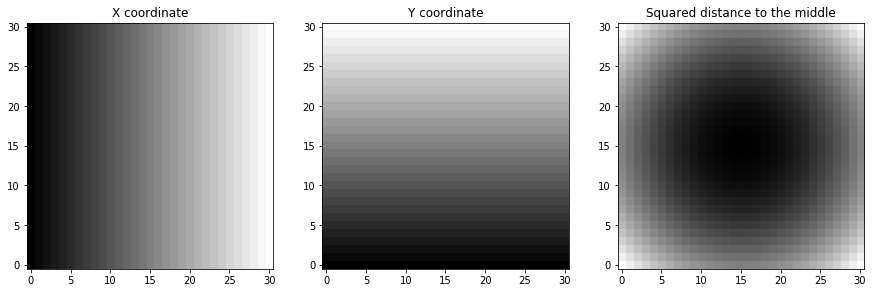And threshold it:

In :
disk = distance <= 15.5**2  # Disk = mask of points which are close to (15,15)
x_0 = extract_points(disk)  # Extract the points' coordinates

plt.figure(figsize=(8,8))                       # Square blackboard
plt.scatter(x_0[:,0], x_0[:,1], s=9, c="blue")  # Display 'x' as a blue point cloud
plt.imshow(disk, cmap="gray")                   # Display the disk in the background
plt.gca().invert_yaxis()  # The y axis is inverted, according to the "image" convention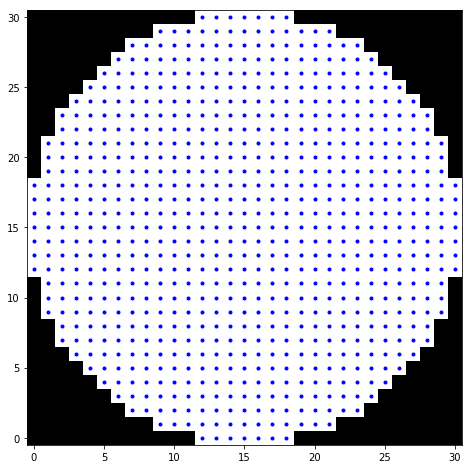Before rescaling the point cloud x_0, making sure that it is centered and has unit diameter:

In :
x_0 = (x_0 - 15)/30  # Center (mean = 0) and normalize (diameter = 1) the point cloud

plt.figure(figsize=(8,8))                       # Square blackboard
plt.scatter(x_0[:,0], x_0[:,1], s=9, c="blue")  # Use blue dots of size 9
plt.axis("equal") ; plt.gca().invert_yaxis()    # The y-axis points towards the bottom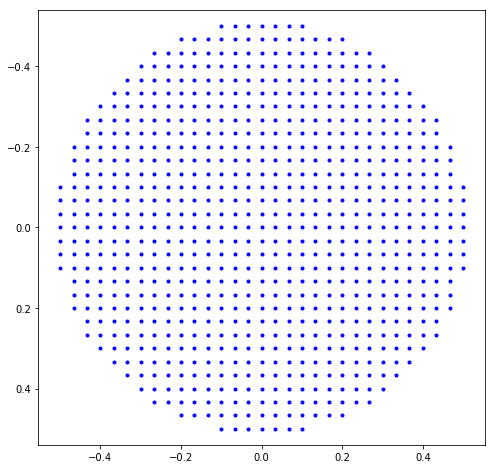That's it! Now, to generate ellipses of arbitrary location and shape, we simply have to use additions and scalings:

In :
def move(x, a,o, w,h):
"""
Stretches and translates a given point cloud 'x'.
The input parameters are the 'abscissa', the 'ordinate',
the 'width' and the 'height' of the final destination.
"""
return x * [w,h] + [a,o]


It works :-)

In :
w = move(x_0, 2,3, 2,4) # center = (2,3), width = 2, height = 4
z = move(x_0, 6,2, 3,2) # center = (6,2), width = 3, height = 2

plt.figure(figsize=(8,8))                         # Square blackboard
plt.scatter(x_0[:,0], x_0[:,1], s=4, c="blue")    # Display 'x_0' using blue dots
plt.scatter(  w[:,0],   w[:,1], s=4, c="orange")  # Display 'w' using orange dots
plt.scatter(  z[:,0],   z[:,1], s=4, c="red")     # Display 'z' using red dots
plt.axis("equal") ; plt.gca().invert_yaxis()   # The y-axis points towards the bottom
plt.title("x_0 (blue), w (orange), z (red)") ;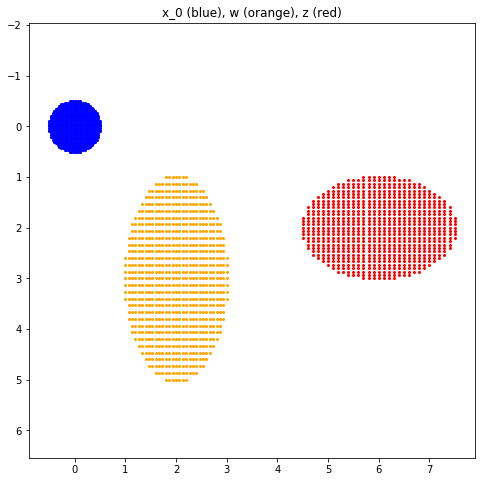### Computing distances between point clouds¶

Ideally, we'd like to find the ellipse that best fits our segmented aorta, thus allowing us to model with explicit parameters the observed subject. We are looking for the absciss a, ordinate o, width w and height h such that x = move(x_0, a,o, w,h) is as close as possible to the "ground truth" data y.

But how should we measure the "error" between the point clouds x and y? The Energy Distance formula, inspired by Newtonian mechanics, provides a decent baseline:

\begin{align} \text{D}(x_1,\dots,x_{\text{N}}~;~y_1,\dots,y_{\text{M}}) ~~ &=~~ \frac{1}{\text{N}\text{M}}\sum_{i=1}^{\text{N}}\sum_{j=1}^{\text{M}} \|x_i-y_j\|\\ ~&-~~ \frac{1}{2\text{N}^2}\sum_{i=1}^{\text{N}}\sum_{j=1}^{\text{N}} \|x_i-x_j\| ~~ - ~~ \frac{1}{2\text{M}^2}\sum_{i=1}^{\text{M}}\sum_{j=1}^{\text{M}} \|y_i-y_j\| \end{align}

Here, $(x_1,\dots,x_{\text{N}})$ and $(y_1,\dots,y_{\text{M}})$ are our "model" and "data" point clouds, while $\|x_i-y_j\|$ denotes the (Euclidean) distance between arbitrary points $x_i$ and $y_j$ in 2D. When the point clouds $x$ and $y$ are restricted to single points $(x_1)$ and $(y_1)$, we simply retrieve the usual distance:

\begin{align} \text{D}(x_1~;~y_1) ~~ &=~~ \frac{1}{\text{1}\cdot\text{1}} \|x_1-y_1\| ~~ -~~ \frac{1}{2\cdot\text{1}^2}\|x_1-x_1\| ~~ - ~~ \frac{1}{2\cdot\text{1}^2} \|y_1-y_1\| \\ &=~~ \|x_1-y_1\|. \end{align}

(Bonus:) Physical interpretation. The quantity $\text{D}(x~;~y)$ can be understood as the potential energy of a configuration of charged particles where the $x_i$'s have a charge $+1/\text{N}$, the $y_j$'s have a charge $-1/\text{M}$, and where the standard Coulombic decay in $1/\|x_i-y_j\|^2$ of the elecric force is replaced by a constant norm vector:

\begin{align} \overrightarrow{\text{F}}_{x_i\rightarrow y_j}~=~ -\frac{1}{\text{NM}}\frac{x_i-y_j}{\|x_i-y_j\|}. \end{align}

This formula is nonnegative in the general case, and null if and only if there is a perfect overlap between the two point clouds. Most importantly, it can be computed with five lines of code:

In :
from scipy.spatial import distance_matrix  # Fills arrays with ‖x_i-y_j‖'s

def D(x, y) :
"""
Computes the 'Energy Distance' between two point clouds.
"""
N, M = len(x), len(y)  # Number of rows=points in 'x' and 'y'
XY = distance_matrix(x,y).sum() / (N*M)  # = (1/NM) * ∑_ij ‖x_i-y_j‖
XX = distance_matrix(x,x).sum() / (N*N)  # = (1/NN) * ∑_ij ‖x_i-x_j‖
YY = distance_matrix(y,y).sum() / (M*M)  # = (1/MM) * ∑_ij ‖y_i-y_j‖
return XY - (XX+YY)/2                    # See the equation above


Note that this simple choice is far from being "optimal": other metrics can very well be preferred in real-life applications. But for today, it is more than good enough: as evidenced here, pairwise distances between the ellipses displayed above match our intuition.

In :
plt.figure(figsize=(8,8))                         # Square blackboard
plt.scatter(x_0[:,0], x_0[:,1], s=4, c="blue")    # Display 'x_0' using blue dots
plt.scatter(  w[:,0],   w[:,1], s=4, c="orange")  # Display 'w' using orange dots
plt.scatter(  z[:,0],   z[:,1], s=4, c="red")     # Display 'z' using red dots
plt.axis("equal") ; plt.gca().invert_yaxis()   # The y-axis points towards the bottom
plt.title("x_0 (blue), w (orange), z (red)") ; plt.show()

print( "Blue-Blue   : {:2.3f}".format(D(x_0,x_0)) )
print( "Blue-Orange : {:2.3f}".format(D(x_0,w)) )
print( "Blue-Red    : {:2.3f}".format(D(x_0,z)) )
print( "Orange-Red  : {:2.3f}".format(D(w,z)) )Blue-Blue   : 0.000
Blue-Orange : 2.738
Blue-Red    : 5.532
Orange-Red  : 2.974


Exercise: Playing around with the functions move and D, convince yourself that the Energy Distance is, indeed, null if and only if there is a perfect overlap between the source and target point clouds.

In :
# Your turn!
# =================

x = move(x_0, 1,2, 4,1)  # abscissa, ordinate, width, height
z = move(x_0, 3,1, 2,3)  # abscissa, ordinate, width, height

# =================

error = D(x, z)
print("Distance from the model 'x' to the target 'z': {:2.3f}".format(error))

plt.figure(figsize=(10,10))                    # Create a square blackboard
plt.scatter(z[:,0], z[:,1], s=9, c="red")      # Display the target 'z' in red
plt.scatter(x[:,0], x[:,1], s=9, c="orange")   # Display the model 'x' in orange
plt.axis("equal") ; plt.gca().invert_yaxis() ;

Distance from the model 'x' to the target 'z': 1.216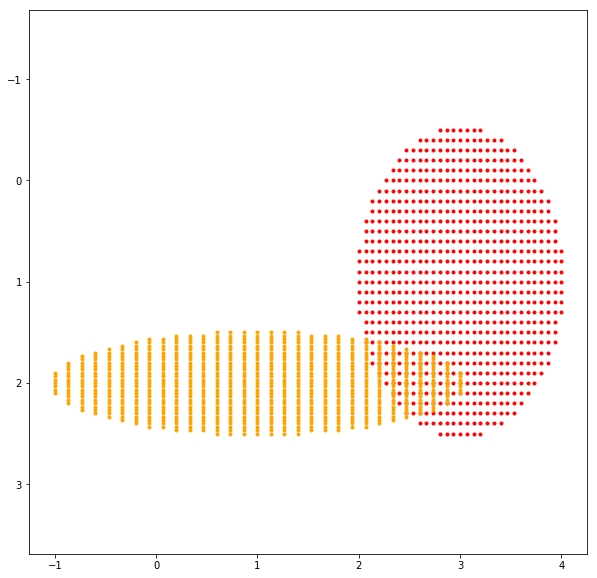### Shape registration¶

In this notebook, we've seen:

• How to segment an aorta from an axial slice, and represent it as a point cloud.
• How to generate simple anatomical models by deforming a reference template shape.
• How to quantify the approximation error with simple mathematical formulas.

Exercise: Now, please try to fit an ellipse x to the aorta y segmented above!

In :
# Your turn!
# =================

x = move(x_0, 100,100, 30,10)  # abscissa, ordinate, width, height

# =================

error = D(x, y)
print("Distance from the model 'x' to the target 'y': {:2.3f}".format(error))

plt.figure(figsize=(10,10))                    # Create a square blackboard
plt.scatter(y[:,0], y[:,1], s=9, c="red")      # Display the target 'y' in red
plt.scatter(x[:,0], x[:,1], s=9, c="orange")   # Display the model 'x' in orange
plt.imshow(I, cmap="gray")                     # Display the slice in the background
plt.axis("equal") ; plt.axis([50,500,50,500])  # Crop the view in a given range
plt.gca().invert_yaxis()

Distance from the model 'x' to the target 'y': 241.035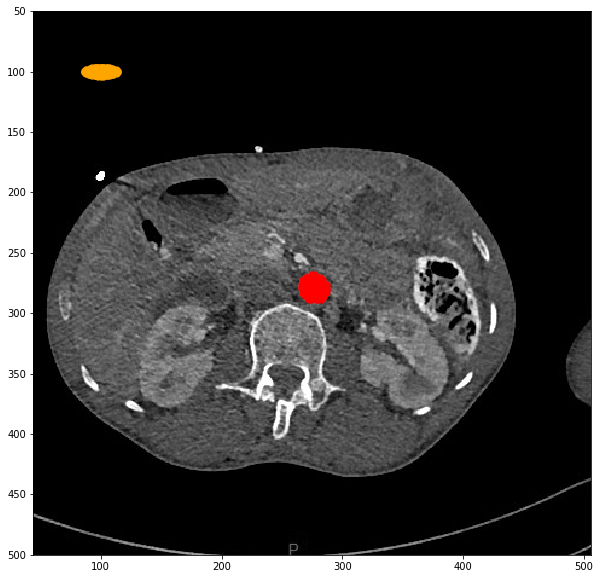Solution: We use simple statistical routines provided by the numpy library: mean and std.

In :
# Your turn!
# =================
a,o =  y.mean(0)  # Mean along the first axis '0' = on each column of 'y'
w,h = 4*y.std(0)  # Standard deviation on each column of 'y'
x = move(x_0, a,o, w,h)  # This is a decent rule of thumb.
# In Part 5, we'll see how to go further.
# =================

error = D(x, y)
print("Distance from the model 'x' to the target 'y': {:2.3f}".format(error))

plt.figure(figsize=(10,10))                    # Create a square blackboard
plt.scatter(y[:,0], y[:,1], s=9, c="red")      # Display the target 'y' in red
plt.scatter(x[:,0], x[:,1], s=9, c="orange")   # Display the model 'x' in orange
plt.imshow(I, cmap="gray")                     # Display the slice in the background
plt.axis("equal") ; plt.axis([250,300,250,300])  # Crop the view in a given range
plt.gca().invert_yaxis()

Distance from the model 'x' to the target 'y': 0.005